# From decision trees to random forests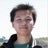Lecturer at School of Information and Communication Technology - Hanoi University of Science and Technology à Giasan.vn - real estate experts
2 Sep 2015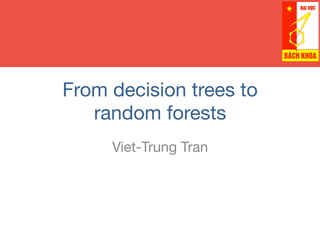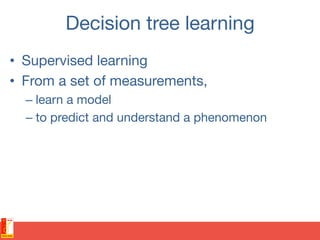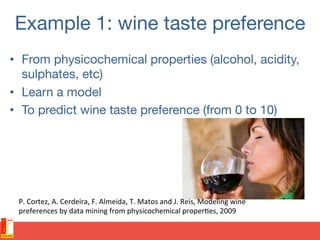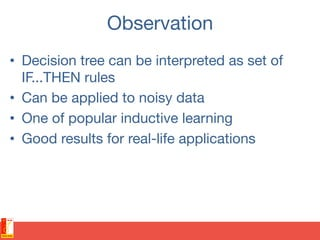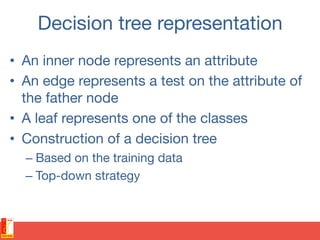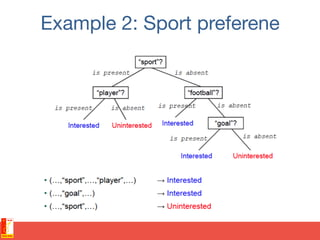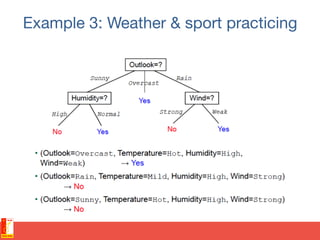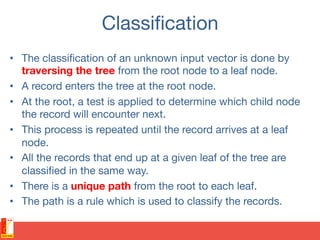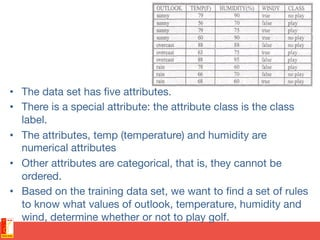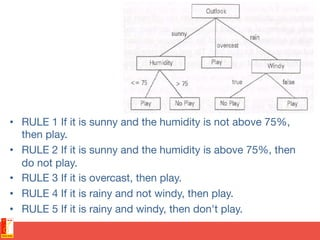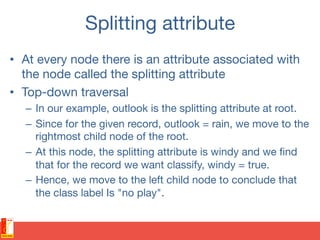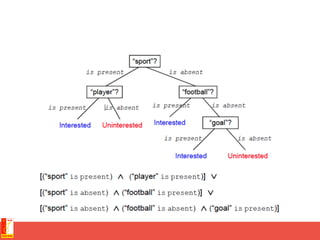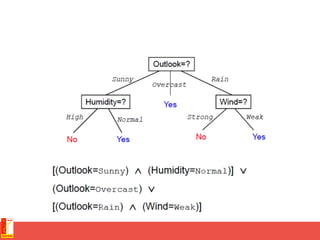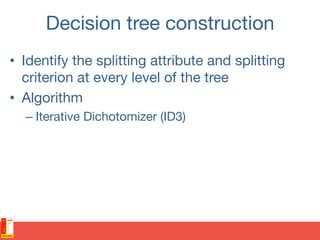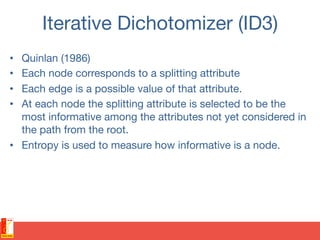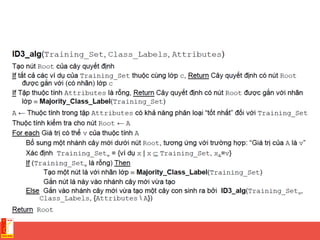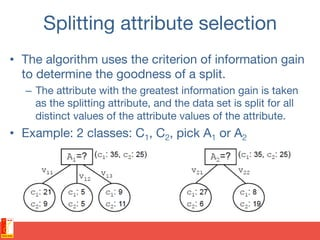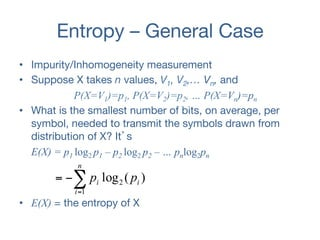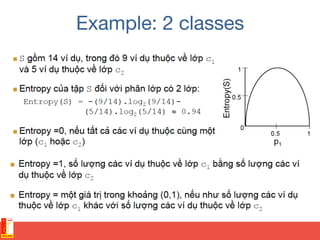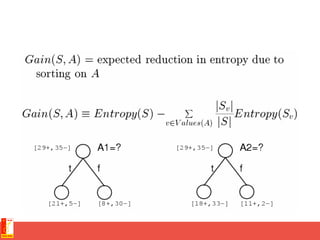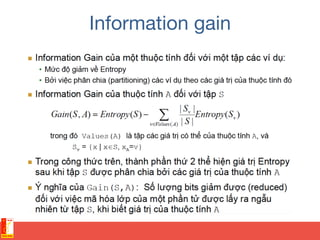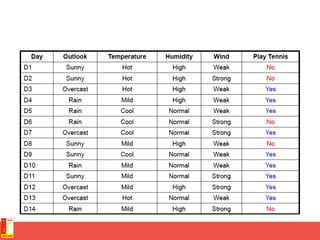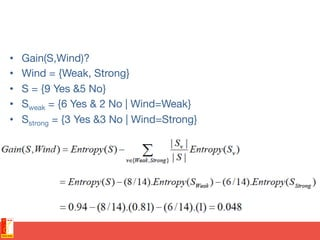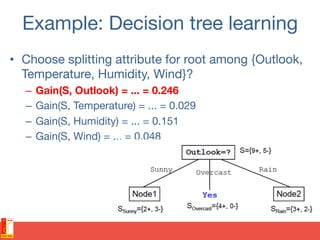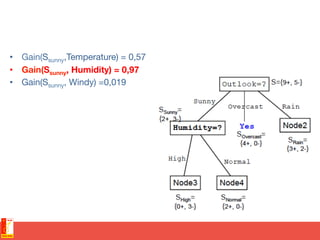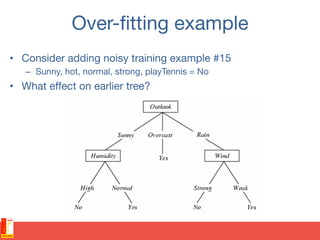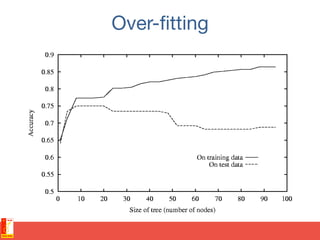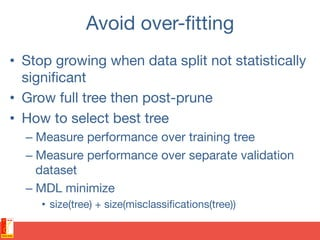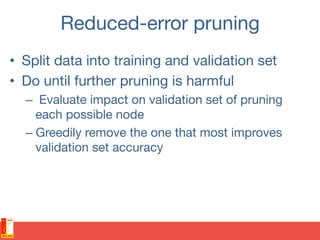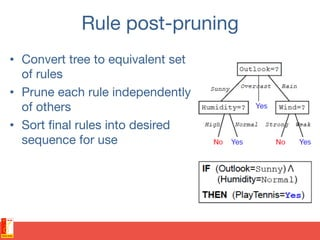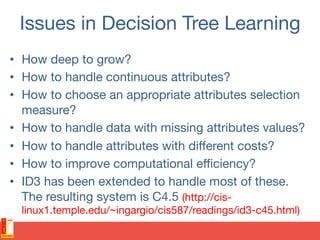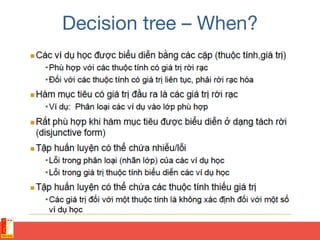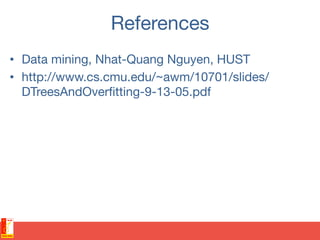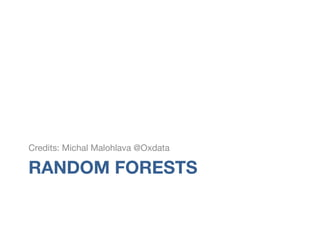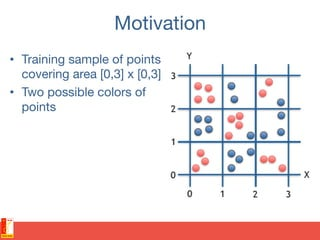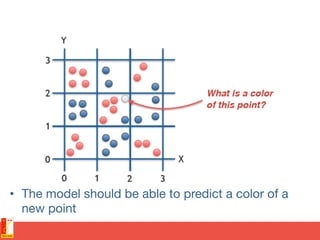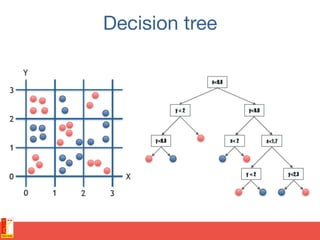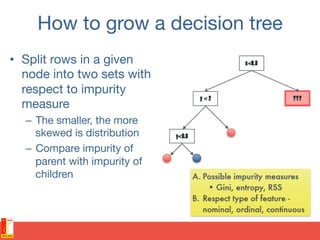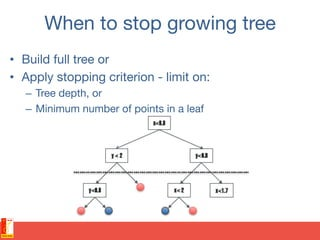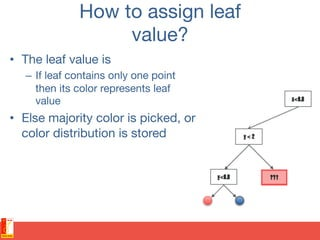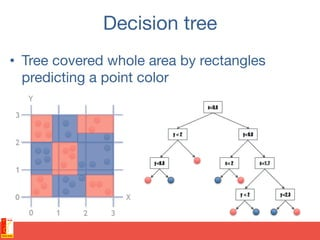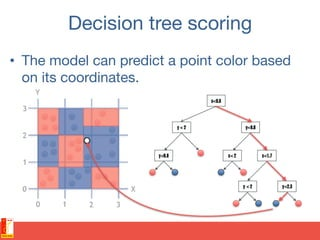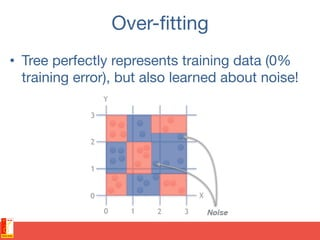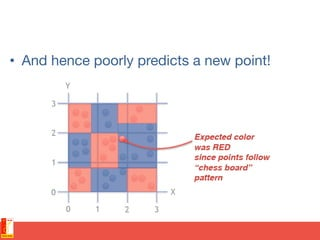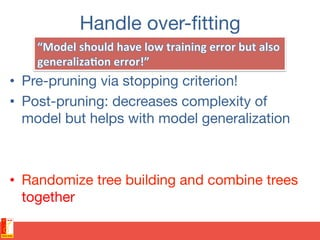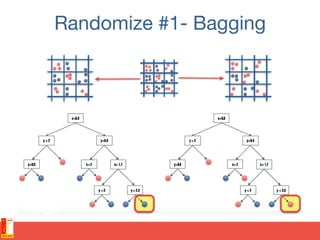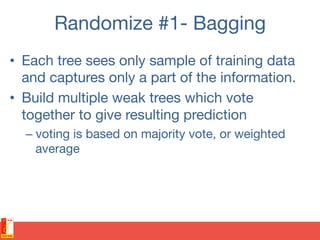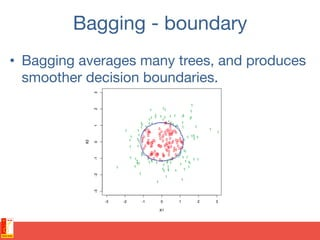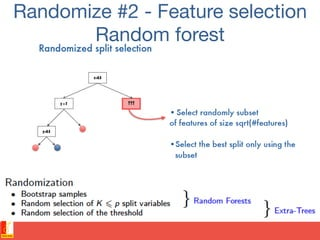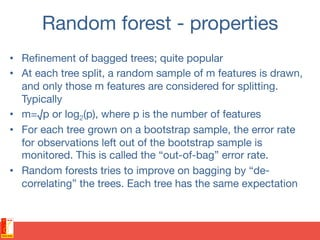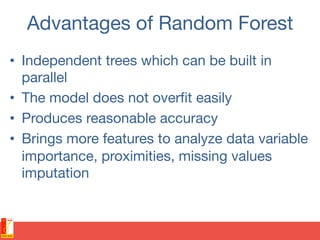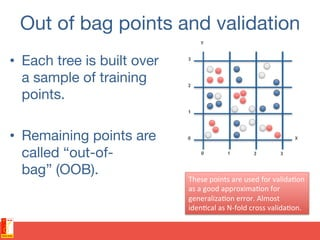1 sur 53

### From decision trees to random forests

• 1. From decision trees to random forests Viet-Trung Tran
• 2. Decision tree learning •  Supervised learning •  From a set of measurements, – learn a model – to predict and understand a phenomenon
• 3. Example 1: wine taste preference •  From physicochemical properties (alcohol, acidity, sulphates, etc) •  Learn a model •  To predict wine taste preference (from 0 to 10) P.  Cortez,  A.  Cerdeira,  F.  Almeida,  T.  Matos  and  J.  Reis,  Modeling  wine   preferences  by  data  mining  from  physicochemical  proper@es,  2009
• 4. Observation •  Decision tree can be interpreted as set of IF...THEN rules •  Can be applied to noisy data •  One of popular inductive learning •  Good results for real-life applications
• 5. Decision tree representation •  An inner node represents an attribute •  An edge represents a test on the attribute of the father node •  A leaf represents one of the classes •  Construction of a decision tree – Based on the training data – Top-down strategy
• 6. Example 2: Sport preferene
• 7. Example 3: Weather & sport practicing
• 8. Classiﬁcation •  The classiﬁcation of an unknown input vector is done by traversing the tree from the root node to a leaf node. •  A record enters the tree at the root node. •  At the root, a test is applied to determine which child node the record will encounter next. •  This process is repeated until the record arrives at a leaf node. •  All the records that end up at a given leaf of the tree are classiﬁed in the same way. •  There is a unique path from the root to each leaf. •  The path is a rule which is used to classify the records.
• 9. •  The data set has ﬁve attributes. •  There is a special attribute: the attribute class is the class label. •  The attributes, temp (temperature) and humidity are numerical attributes •  Other attributes are categorical, that is, they cannot be ordered. •  Based on the training data set, we want to ﬁnd a set of rules to know what values of outlook, temperature, humidity and wind, determine whether or not to play golf.
• 10. •  RULE 1 If it is sunny and the humidity is not above 75%, then play. •  RULE 2 If it is sunny and the humidity is above 75%, then do not play. •  RULE 3 If it is overcast, then play. •  RULE 4 If it is rainy and not windy, then play. •  RULE 5 If it is rainy and windy, then don't play.
• 11. Splitting attribute •  At every node there is an attribute associated with the node called the splitting attribute •  Top-down traversal –  In our example, outlook is the splitting attribute at root. –  Since for the given record, outlook = rain, we move to the rightmost child node of the root. –  At this node, the splitting attribute is windy and we ﬁnd that for the record we want classify, windy = true. –  Hence, we move to the left child node to conclude that the class label Is "no play".
• 14. Decision tree construction •  Identify the splitting attribute and splitting criterion at every level of the tree •  Algorithm – Iterative Dichotomizer (ID3)
• 15. Iterative Dichotomizer (ID3) •  Quinlan (1986) •  Each node corresponds to a splitting attribute •  Each edge is a possible value of that attribute. •  At each node the splitting attribute is selected to be the most informative among the attributes not yet considered in the path from the root. •  Entropy is used to measure how informative is a node.
• 17. Splitting attribute selection •  The algorithm uses the criterion of information gain to determine the goodness of a split. –  The attribute with the greatest information gain is taken as the splitting attribute, and the data set is split for all distinct values of the attribute values of the attribute. •  Example: 2 classes: C1, C2, pick A1 or A2
• 18. Entropy – General Case •  Impurity/Inhomogeneity measurement •  Suppose X takes n values, V1, V2,… Vn, and P(X=V1)=p1, P(X=V2)=p2, … P(X=Vn)=pn •  What is the smallest number of bits, on average, per symbol, needed to transmit the symbols drawn from distribution of X? It’s E(X) = p1 log2 p1 – p2 log2 p2 – … pnlog2pn •  E(X) = the entropy of X )(log 1 2 i n i i pp∑= −=
• 23. •  Gain(S,Wind)? •  Wind = {Weak, Strong} •  S = {9 Yes &5 No} •  Sweak = {6 Yes & 2 No | Wind=Weak} •  Sstrong = {3 Yes &3 No | Wind=Strong}
• 24. Example: Decision tree learning •  Choose splitting attribute for root among {Outlook, Temperature, Humidity, Wind}? –  Gain(S, Outlook) = ... = 0.246 –  Gain(S, Temperature) = ... = 0.029 –  Gain(S, Humidity) = ... = 0.151 –  Gain(S, Wind) = ... = 0.048
• 25. •  Gain(Ssunny,Temperature) = 0,57 •  Gain(Ssunny, Humidity) = 0,97 •  Gain(Ssunny, Windy) =0,019
• 26. Over-ﬁtting example •  Consider adding noisy training example #15 –  Sunny, hot, normal, strong, playTennis = No •  What eﬀect on earlier tree?
• 28. Avoid over-ﬁtting •  Stop growing when data split not statistically signiﬁcant •  Grow full tree then post-prune •  How to select best tree – Measure performance over training tree – Measure performance over separate validation dataset – MDL minimize •  size(tree) + size(misclassiﬁcations(tree))
• 29. Reduced-error pruning •  Split data into training and validation set •  Do until further pruning is harmful –  Evaluate impact on validation set of pruning each possible node – Greedily remove the one that most improves validation set accuracy
• 30. Rule post-pruning •  Convert tree to equivalent set of rules •  Prune each rule independently of others •  Sort ﬁnal rules into desired sequence for use
• 31. Issues in Decision Tree Learning •  How deep to grow? •  How to handle continuous attributes? •  How to choose an appropriate attributes selection measure? •  How to handle data with missing attributes values? •  How to handle attributes with diﬀerent costs? •  How to improve computational eﬃciency? •  ID3 has been extended to handle most of these. The resulting system is C4.5 (http://cis- linux1.temple.edu/~ingargio/cis587/readings/id3-c45.html)
• 33. References •  Data mining, Nhat-Quang Nguyen, HUST •  http://www.cs.cmu.edu/~awm/10701/slides/ DTreesAndOverﬁtting-9-13-05.pdf
• 34. RANDOM FORESTS Credits: Michal Malohlava @Oxdata
• 35. Motivation •  Training sample of points covering area [0,3] x [0,3] •  Two possible colors of points
• 36. •  The model should be able to predict a color of a new point
• 38. How to grow a decision tree •  Split rows in a given node into two sets with respect to impurity measure –  The smaller, the more skewed is distribution –  Compare impurity of parent with impurity of children
• 39. When to stop growing tree •  Build full tree or •  Apply stopping criterion - limit on: –  Tree depth, or –  Minimum number of points in a leaf
• 40. How to assign leaf  value? •  The leaf value is –  If leaf contains only one point then its color represents leaf value •  Else majority color is picked, or color distribution is stored
• 41. Decision tree •  Tree covered whole area by rectangles predicting a point color
• 42. Decision tree scoring •  The model can predict a point color based on its coordinates.
• 43. Over-ﬁtting •  Tree perfectly represents training data (0% training error), but also learned about noise!
• 44. •  And hence poorly predicts a new point!
• 45. Handle over-ﬁtting •  Pre-pruning via stopping criterion! •  Post-pruning: decreases complexity of model but helps with model generalization •  Randomize tree building and combine trees together
• 48. Randomize #1- Bagging •  Each tree sees only sample of training data and captures only a part of the information. •  Build multiple weak trees which vote together to give resulting prediction – voting is based on majority vote, or weighted average
• 49. Bagging - boundary •  Bagging averages many trees, and produces smoother decision boundaries.
• 50. Randomize #2 - Feature selection  Random forest
• 51. Random forest - properties •  Reﬁnement of bagged trees; quite popular •  At each tree split, a random sample of m features is drawn, and only those m features are considered for splitting. Typically •  m=√p or log2(p), where p is the number of features •  For each tree grown on a bootstrap sample, the error rate for observations left out of the bootstrap sample is monitored. This is called the “out-of-bag” error rate. •  Random forests tries to improve on bagging by “de- correlating” the trees. Each tree has the same expectation
• 52. Advantages of Random Forest •  Independent trees which can be built in parallel •  The model does not overﬁt easily •  Produces reasonable accuracy •  Brings more features to analyze data variable importance, proximities, missing values imputation
• 53. Out of bag points and validation •  Each tree is built over a sample of training points. •  Remaining points are called “out-of- bag” (OOB). These  points  are  used  for  valida@on   as  a  good  approxima@on  for   generaliza@on  error.  Almost   iden@cal  as  N-­‐fold  cross  valida@on.